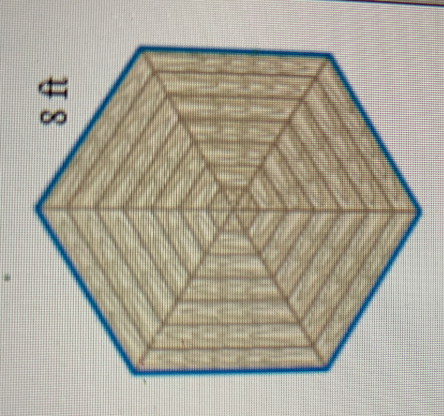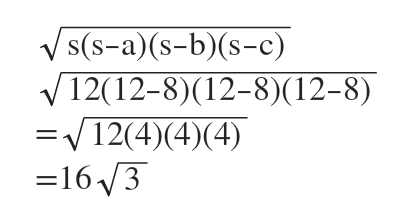Question
4 views

Luis plans to build a gazebo with a concrete floor shaped like a regular hexagon.  Each side will measure 8 feet as shown in the diagram and the floor will be 6 inches deep. How many cubic feet of concrete are needed? Assume that Luis cannot buy a fraction of the cubic foot.

(Hint: Fivide the hexagon into equilateral triangles and use Herons formula to find the area of the top surface of the hexagon)

check_circle

Step 1We find the area of the hexagon first.

It has 6 equilateral triangles.

Each triangle has a side length of 8 ft.

Step 2

Half perimeter of a triangle = (8+8+8)/2=12

Using Heron's formula to find the area of a triangle.So area of 6 triangles = 6*16sqrt(3)= 96sqrt(3) square foot.

...

### Want to see the full answer?

See Solution

#### Want to see this answer and more?

Solutions are written by subject experts who are available 24/7. Questions are typically answered within 1 hour.*

See Solution
*Response times may vary by subject and question.
Tagged in